Mathematics Commons™

17,860 Full-Text Articles 17,114 Authors 4,137,002 Downloads285 Institutions

All Articles in Mathematics

17,860 full-text articles. Page 461 of 528.

Noncomputable Functions In The Blub-Shub-Smale Model, 2011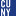Southern Illinois University

Noncomputable Functions In The Blub-Shub-Smale Model, Wesley Calvert, Ken Kramer, Russell Miller

Publications and Research

Working in the Blum-Shub-Smale model of computation on the real numbers, we answer several questions of Meer and Ziegler. First, we show that, for each natural number d, an oracle for the set of algebraic real numbers of degree at most d is insufficient to allow an oracle BSS-machine to decide membership in the set of algebraic numbers of degree d + 1. We add a number of further results on relative computability of these sets and their unions. Then we show that the halting problem for BSS-computation is not decidable below any countable oracle set, and give a more specific ...

Darboux Integrability - A Brief Historial Survey, 2011Utah State University

Darboux Integrability - A Brief Historial Survey, Ian M. Anderson

Mathematics and Statistics Faculty Presentations

No abstract provided.

Mathematical Techniques For Assigning First-Year Seminars, 2011Dickinson College

Mathematical Techniques For Assigning First-Year Seminars, Thanh Thien To

Student Honors Theses By Year

Every first-year student at Dickinson College is required to take a first-year seminar. The summer before the students arrive they each select six seminars among all the seminar choices. Each student is then assigned to a seminar from their list, if possible. Currently, this process is performed manually, which is tedious and time consuming. This research is concerned with utilizing mathematical techniques to assign first-year students to seminars. Specifically, we develop a technique that not only assigns students to seminars, but also seeks to balance the gender ratio and international student ratio of the students in the classes. In addition ...

Mind The Gap: How Metaphor And Mathematics Make (And Remake) The World, 2011Skidmore College

Mind The Gap: How Metaphor And Mathematics Make (And Remake) The World, Susan J. Sechrist

Master of Arts in Liberal Studies (MALS) Student Scholarship

To challenge myself beyond writing an analytical research paper, I decided that the best way to encapsulate my interdisciplinary experience was to do an interdisciplinary project. Since my area of focus was about the relationship between fiction and nonfiction, my thesis would be as well. This project consists of several sections of analytical work with chapters from an ongoing work of fiction sandwiched (I hope, illustratively) between. The story, called The Bathymetrist, is about Milo Finn, a teenaged girl who spends summer days in a row boat out on a pond behind her house. She begins to map the bottom ...

2011Illinois Wesleyan University

Generalized Exponential Euler Polynomials And Exponential Splines, Tian-Xiao He

Tian-Xiao He

Here presented is constructive generalization of exponential Euler polynomial and exponential splines based on the interrelationship between the set of concepts of Eulerian polynomials, Eulerian numbers, and Eulerian fractions and the set of concepts related to spline functions. The applications of generalized exponential Euler polynomials in series transformations and expansions are also given.

Linear Algebra, 2011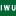Illinois Wesleyan University

Linear Algebra, Lawrence Stout

Scholarship

This book is designed to deal with all of the concepts of linear algebra first in R2, a simple context where algorithmic concerns are minimized and geometric intuition can be brought to bear. Then those same concepts are dealt with in full generality. I find that this conceptual front loading helps in the understanding of the rest of the material. Students can see easily what aspects of the plane are focused on when we think of it as a vector space; linear transformations can be understood as deformations of the plane of a very special type. Nothing more complicated than ...

Linear Algebra, 2011Illinois Wesleyan University

Linear Algebra, Lawrence Stout

Lawrence N. Stout

This book is designed to deal with all of the concepts of linear algebra first in R2, a simple context where algorithmic concerns are minimized and geometric intuition can be brought to bear. Then those same concepts are dealt with in full generality. I find that this conceptual front loading helps in the understanding of the rest of the material. Students can see easily what aspects of the plane are focused on when we think of it as a vector space; linear transformations can be understood as deformations of the plane of a very special type. Nothing more complicated than ...

Moduli Problems In Derived Noncommutative Geometry, 2011University of Pennsylvania

Moduli Problems In Derived Noncommutative Geometry, Pranav Pandit

Publicly Accessible Penn Dissertations

We study moduli spaces of boundary conditions in 2D topological field theories. To a compactly generated linear infinity-category X, we associate a moduli functor M_X parametrizing compact objects in X. The Barr-Beck-Lurie monadicity theorem allows us to establish the descent properties of M_X, and show that M_X is a derived stack. The Artin-Lurie representability criterion makes manifest the relation between finiteness conditions on X, and the geometricity of M_X. If X is fully dualizable (smooth and proper), then M_X is geometric, recovering a result of Toën-Vaquie from a new perspective. Properness of X does not imply geometricity in general: perfect ...

Equivariant Tensors On Polar Manifolds, 2011University of Pennsylvania

Equivariant Tensors On Polar Manifolds, Ricardo Augusto E. Mendes

Publicly Accessible Penn Dissertations

EQUIVARIANT TENSORS ON POLAR MANIFOLDS
Ricardo Mendes
This PhD dissertation has two parts, both dealing with extension questions for equivariant
tensors on a polar G-manifold M with section Σ ⊂ M.
Chapter 3 contains the first part, regarding the so-called smoothness conditions: If a
tensor defined only along Σ is equivariant under the generalized Weyl group W (Σ), then it exends to a G-equivariant tensor on M if and only if it satisfies the smoothness conditions. The main result is stated and proved in the first section, and an algorithm is also provided that calculates smoothness conditions.
Chapter ...

2011University of Pennsylvania

The Classification Of Simply Connected Biquotients Of Dimension At Most 7 And 3 New Examples Of Almost Positively Curved Manifolds, Jason Devito

Publicly Accessible Penn Dissertations

We classify all compact 1-connected manifolds $M^n$ for $2 \leq n leq 7$ which are
diffeomorphic to biquotients. Further, given that $M$ is diffeomorphic to a biquotient,
we classify the biquotients it is diffeomorphic to. Finally, we show the homogeneous
space $Sp(3)\Sp(1) \tines Sp(1)$ and two of its quotients $Sp(3)\Sp(1) \times Sp(1) \times S^1$ and
$\delta S^1 \backslash Sp(3)/Sp(1)\times Sp(1)$ admit metrics of almost positive curvature.
iv

Homological Projective Duality For Gr(3,6), 2011University of Pennsylvania

Homological Projective Duality For Gr(3,6), Dragos Deliu

Publicly Accessible Penn Dissertations

Homological Projective Duality is a homological extension of the classical no-

tion of projective duality. Constructing the homological projective dual of a variety

allows one to describe semiorthogonal decompositions on the bounded derived cat-

egory of coherent sheaves for all the complete linear sections of the initial variety.

This gives a powerful method to construct decompositions for a big class of varieties,

however examples for which this duality is understood are very few.

In this thesis we investigate the case of Gr(3, 6) with respect to the Plucker

embedding.

The Derived Category And The Singularity Category, 2011University of Pennsylvania

The Derived Category And The Singularity Category, Mehmet U. Isik

Publicly Accessible Penn Dissertations

We prove an equivalence between the derived category of a variety and the equivari- ant/graded singularity category of a corresponding singular variety. The equivalence also holds at the dg level.

2011University of Pennsylvania

The Higher Riemann-Hilbert Correspondence And Multiholomorphic Mappings, Aaron M. Smith

Publicly Accessible Penn Dissertations

This thesis consists in two chapters. In the first part we describe an $A_\infty$-quasi-equivalence of dg-categories between Block's $\Perf$, corresponding to the de Rham dga $\As$ of a compact manifold $M$ and the dg-category of infinity-local systems on $M$. We understand this as a generalization of the Riemann-Hilbert correspondence to $\Z$-graded connections (superconnections in some circles). In one formulation an infinity-local system is an $(\infty,1)$-functor between the $(\infty,1)$-categories ${\pi}_{\infty}M$ and a repackaging of the dg-category of cochain complexes by virtue of the simplicial nerve and Dold-Kan. This theory makes ...

2011University of Pennsylvania

The Classification Of Automorphism Groups Of Rational Elliptic Surfaces With Section, Tolga Karayayla

Publicly Accessible Penn Dissertations

In this dissertation, we give a classification of (regular) automorphism groups of relatively minimal rational elliptic surfaces with section over the field ℂ which have non-constant J-maps. The automorphism group Aut(B) of such a surface B is the semi-direct product of its Mordell-Weil group MW(B) and the subgroup Autσ(B) of the automorphisms preserving the zero section σ of the rational elliptic surface B. The configuration of singular fibers on the surface determines the Mordell-Weil group as has been shown by Oguiso and Shioda, and Autσ(B) also depends on the singular fibers. The classification ...

Viscosity Of Bacterial Suspensions: Hydrodynamic Interactions And Self-Induced Noise, 2011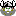Cleveland State University

Viscosity Of Bacterial Suspensions: Hydrodynamic Interactions And Self-Induced Noise, Shawn D. Ryan, Brian M. Haines, Leonid Berlyand, Falko Ziebert, Igor S. Aranson

Mathematics Faculty Publications

The viscosity of a suspension of swimming bacteria is investigated analytically and numerically. We propose a simple model that allows for efficient computation for a large number of bacteria. Our calculations show that long-range hydrodynamic interactions, intrinsic to self-locomoting objects in a viscous fluid, result in a dramatic reduction of the effective viscosity. In agreement with experiments on suspensions of Bacillus subtilis, we show that the viscosity reduction is related to the onset of large-scale collective motion due to interactions between the swimmers. The simulations reveal that the viscosity reduction occurs only for relatively low concentrations of swimmers: Further increases ...

The Falc-Loop Web Server For Protein Loop Modeling, 2011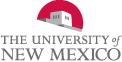University of New Mexico

The Falc-Loop Web Server For Protein Loop Modeling, Evangelos A. Coutsias, Juns Ko, Dongseon Lee, Hahnbeom Park, Julian Lee, Chaok Seok

Faculty and Staff Publications

The FALC-Loop web server provides an online interface for protein loop modeling by employing an ab initio loop modeling method called FALC (fragment assembly and analytical loop closure). The server may be used to construct loop regions in homology modeling, to refine unreliable loop regions in experimental structures or to model segments of designed sequences. The FALC method is computationally less expensive than typical ab initio methods because the conformational search space is effectively reduced by the use of fragments derived from a structure database. The analytical loop closure algorithm allows efficient search for loop conformations that fit into the ...

Algorithms For Bivariate Singularity Analysis, 2011University of Pennsylvania

Algorithms For Bivariate Singularity Analysis, Timothy Devries

Publicly Accessible Penn Dissertations

An algorithm for bivariate singularity analysis is developed. For a wide class of bivariate, rational functions F = P/Q, this algorithm produces rigorous numerics for the asymptotic analysis of the Taylor coefficients of F at the origin. The paper begins with a self-contained treatment of multivariate singularity analysis. The analysis itself relies heavily on the geometry of the pole set VQ of F with respect to a height function h. This analysis is then applied to obtain asymptotics for the number of bicolored supertrees, computed in a purely multivariate way. This example is interesting in that the asymptotics can not ...

Heegaard Floer Invariants And Cabling, 2011University of Pennsylvania

Heegaard Floer Invariants And Cabling, Jennifer Hom

Publicly Accessible Penn Dissertations

A natural question in knot theory is to ask how certain properties of a knot behave under satellite operations. We will focus on the satellite operation of cabling, and on Heegaard Floer-theoretic properties. In particular, we will give a formula for the Ozsvath-Szabo concordance invariant tau of iterated cables of a knot K in terms of the cabling parameters, tau(K), and a new concordance invariant, epsilon(K). We show that, in many cases, varepsilon gives better bounds on the 4-ball genus of a knot that tau alone, and discuss further applications of epsilon. We will also completely classify when ...

The Quantum Dialectic, 2011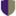Pitzer College

The Quantum Dialectic, Logan Kelley

Pitzer Senior Theses

A philosophic account of quantum physics. The thesis is divided into two parts. Part I is dedicated to laying the groundwork of quantum physics, and explaining some of the primary difficulties. Subjects of interest will include the principle of locality, the quantum uncertainty principle, and Einstein's criterion for reality. Quantum dilemmas discussed include the double-slit experiment, observations of spin and polarization, EPR, and Bell's theorem. The first part will argue that mathematical-physical descriptions of the world fall short of explaining the experimental observations of quantum phenomenon. The problem, as will be argued, is framework of the physical descriptive ...

2011Georgia State University

Three Topics In Analysis: (I) The Fundamental Theorem Of Calculus Implies That Of Algebra, (Ii) Mini Sums For The Riesz Representing Measure, And (Iii) Holomorphic Domination And Complex Banach Manifolds Similar To Stein Manifolds, Panakkal J. Mathew

Mathematics Dissertations

We look at three distinct topics in analysis. In the first we give a direct and easy proof that the usual Newton-Leibniz rule implies the fundamental theorem of algebra that any nonconstant complex polynomial of one complex variable has a complex root. Next, we look at the Riesz representation theorem and show that the Riesz representing measure often can be given in the form of mini sums just like in the case of the usual Lebesgue measure on a cube. Lastly, we look at the idea of holomorphic domination and use it to define a class of complex Banach manifolds ...